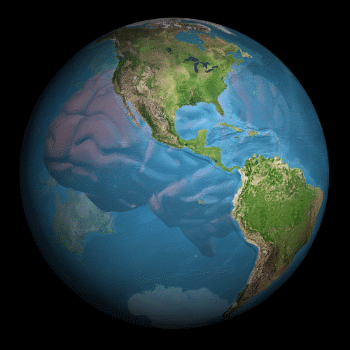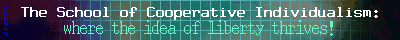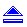.SCI LIBRARY## The Natural Rate of Interest

### Fred Foldvary

The Austrian economist Eugen von Bohm-Bawerk refuted the fructification and reproductive theories of interest held by Henry George and others.

Bohm-Bawerk argued that if the interest rate were zero, then seeds would sell for as much as grown plants (minus the labor needed to grow them). If grown plants had a higher price, it would be profitable to buy the seeds, so the price of seeds would be bid up until the profit was gone. So the greater value of grown plants over seeds does not determine the rate of interest, since the effect would be rather to bid up the price of seeds.

Alas, Bohm-Bawerk omitted the land-rent cost of moving through time. When you work out the math, if the interest rate, "i", falls to zero, it raises land rent, which is also part of the cost of passing through time, so the price of seeds does not rise to equal the harvest value of timber (which would be absurd, obviously). Foresters had already worked this out, in what was and is called the "Faustmann Formula." Glad to supply details, or refer you to my monograph, Concepts of Financial Maturity of Timber.

Austrian economists instead presented the time-preference theory of interest, in which it is the preference for present-day goods over future goods that creates a discount in the future, which is the rate of interest. This became accepted by mainstream theory, to some degree, as expressed by Irving Fisher in his landmark work, The Theory of Interest (1930). George at least recognized that pure interest has to do with time, and that it is a natural market phenomenon and not a problem.

#### The Austrian Response

The Austrian economist Eugen von Bohm-Bawerk refuted the fructification and reproductive theories of interest held by Henry George and others. Bohm-Bawerk argued that if the interest rate were zero, then seeds would sell for as much as grown plants (minus the labor needed to grow them). If grown plants had a higher price, it would be profitable to buy the seeds, so the price of seeds would be bid up until the profit was gone. So the greater value of grown plants over seeds does not determine the rate of interest, since the effect would be rather to bid up the price of seeds.

Alas, Bohm-Bawerk omitted the land-rent cost of moving through time. When you work out the math, if the interest rate, "i", falls to zero, it raises land rent, which is also part of the cost of passing through time, so the price of seeds does not rise to equal the harvest value of timber (which would be absurd, obviously). Foresters had already worked this out, in what was and is called the "Faustmann Formula." Glad to supply details, or refer you to my monograph, Concepts of Financial Maturity of Timber.

Austrian economists instead presented the time-preference theory of interest, in which it is the preference for present-day goods over future goods that creates a discount in the future, which is the rate of interest. This became accepted by mainstream theory, to some degree, as expressed by Irving Fisher in his landmark work, The Theory of Interest (1930). George at least recognized that pure interest has to do with time, and that it is a natural market phenomenon and not a problem.